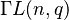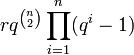# General semilinear group

## Definition

Suppose$R$ is a commutative unital ring and$n$ is a natural number. The general semilinear group of degree$n$ over$R$, denoted$\Gamma L(n,R)$, is defined as the group of all invertible$R$-semilinear transformations from a$n$-dimensional free module over$R$ to itself.

It can also be described explicitly as an external semidirect product of the general linear group$GL(n,R)$ by the automorphism group of$R$ as a commutative unital ring (acting entry-wise on the matrices), i.e.:$\Gamma L(n,R) = GL(n,R) \rtimes \operatorname{Aut}(R)$

### Special case of fields

Suppose$k$ is the prime subfield of$K$ and suppose that$K$ is a Galois extension over$k$ (this is always true for$K$ a finite field). Then,$\operatorname{Aut}(K) = \operatorname{Gal}(K/k)$ and we can rewrite the group as:$\Gamma L(n,K) = GL(n,K) \rtimes \operatorname{Gal}(K/k)$

where the action of$\operatorname{Gal}(K/k)$ on$GL(n,K)$ is obtained by inducing the corresponding Galois automorphism on each matrix entry.

If$q$ is a prime power, we denote by$\Gamma L(n,q)$ the group$\Gamma L(n,\mathbb{F}_q)$ where$\mathbb{F}_q$ is the (unique up to isomorphism) field of size$q$.

## Arithmetic functions

### For a finite field

We consider here a field$K = \mathbb{F}_q$ of size$q = p^r$ where$p$ is the field characteristic, so$r$ is a natural number.

The prime subfield is$k = \mathbb{F}_p$, and the extension$K/k$ has degree$r$. The Galois group of the extension thus has size$r$. Note that the Galois group of the extension is always a cyclic group of order$r$ and is generated by the Frobenius automorphism$x \mapsto x^p$.

We are interested in the group$\Gamma L(n,q)$.

Function Value Explanation
order$rq^{\binom{n}{2}}\prod_{i=1}^n (q^i - 1)$ order of semidirect product is product of orders: we multiply the order$r$ of the Galois group with the order of the general linear group.

## Particular cases

### Finite cases

We consider a field of size$q = p^r$ where$p$ is the underlying prime and field characteristic, and therefore$r$ is the degree of the extension over the prime subfield and also the order of the Galois group.

Note that in the case$r = 1$, the general semilinear group coincides with the general linear group.$q$ (field size)$p$ (underlying prime, field characteristic)$r$ (degree of extension over prime subfield)$n$$\Gamma L(n,q)$ order of$\Gamma L(n,q)$
2 2 1 1 trivial group 1
3 3 1 1 cyclic group:Z2 1
4 2 2 1 symmetric group:S3 6
5 5 1 1 cyclic group:Z4 4
7 7 1 1 cyclic group:Z6 6
8 2 3 1 semidirect product of Z7 and Z3 21
2 2 1 2 symmetric group:S3 6
3 3 1 2 general linear group:GL(2,3) 48
4 2 2 2 general semilinear group:GammaL(2,4) 360
5 5 1 2 general linear group:GL(2,5) 480